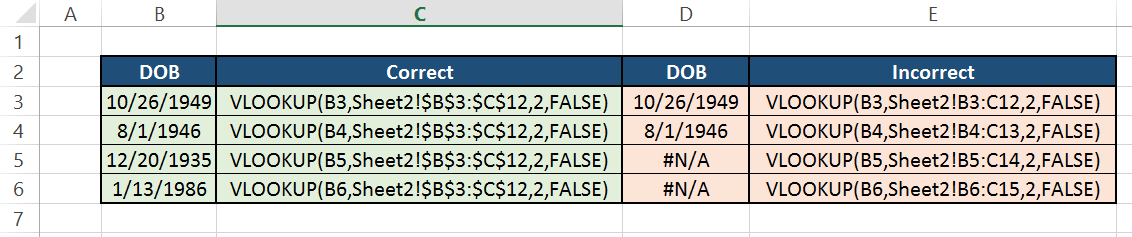# What is a VLOOKUP and HLOOKUP?

These are two excel formulas everyone should know.

VLOOKUP and HLOOKUP

These two functions will definitely be helpful if you are a daily Excel user.  The VLOOKUP function looks for a value in the leftmost column of a table, and then returns a value in the same row from a column you specify.  The HLOOKUP function is very similar, however instead of looking for a value in the leftmost column, it looks for a value in the top row of a table.  The V stands for vertical (returns value from a column), and the H stands for horizontal (returns value from a row).  Both of these formulas can be very handy especially when you are working with large data sets.

Even though the VLOOKUP and HLOOKUP functions differ slightly, they are made up of the same arguments and have the same syntax.  Below is the formula for the VLOOKUP function (if you want to do an HLOOKUP instead just replace the V with an H):

=VLOOKUP(lookup_value, table_array, col_index_num, [range_lookup])

Lookup_value: the value to be found in the first column of the table – can be a value, reference, or text string.

Table_array: the table_array is a table of text, numbers, or logical values in which data is retrieved.   If the table array is horizontal instead of vertical, use the HLOOKUP function.

Col_index_num: this is the column number in table_array from which the matching value should be returned.

Range_lookup: the range_lookup is a logical value and can be set to either TRUE or FALSE.  A value of TRUE will find the closest match in the first column (or row if using the HLOOKUP), and a value of FALSE will find only an exact match.

Example: You have the two spreadsheets below and want to add the DOB information from Sheet 2 into your table in Sheet 1, but do not want to have to manually search for it.  In this instance use the VLOOKUP function to auto-fill the DOB column in Sheet 1.

This slideshow requires JavaScript.

To insert the DOB field from Sheet 2 into Column I of Sheet 1, enter the following VLOOKUP in I1 and drag the formula down.

=VLOOKUP(B3, Sheet2!\$B\$3:\$C\$12, 2, FALSE)

Lookup_value: The TINs in Column B from Sheet 1

Table_array: The table on Sheet 2 (make sure to use the \$ to make the cells an absolute reference)

Col_index_num: 2 – you want to return the second column of the table on Sheet 2

Range_lookup: FALSE – you want to only return a DOB if the TIN is an exact match

If done correctly, Column I should look like this:Stuck?

When you enter in the formula and #N/A appears, then you know something went wrong.  If you receive this error message then check to see if you fell victim to these three mistakes.  The most common reason why a VLOOKUP function could return an error message is because the leftmost column in the reference sheet (Sheet 2 in this example) is not in ascending or alphabetical order.

Another reason why VLOOKUP functions do not work is because the format of the Lookup_value in the main sheet does not match the format in the table array.  It may appear that they are the same, but double check to make sure – one might be in number format and the other as text.

If you check the way the data is sorted and the data format but are still getting an error then make sure that the absolute reference characters (\$) are included within the formula when selecting the table_array.

The giveaway is if the first cell you typed the formula in returns the correct value and most of the cells that you dragged the formula down to fill mostly return an error (see column D below).  Absolute references make it so the selected table_array will not change when the data is copied or filled.Still stuck?